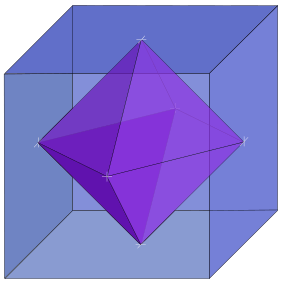# Dual polyhedron facts for kids

Kids Encyclopedia FactsThis diagram shows how the cube and octahedron are dual. Each vertex of the octahedron matches with a face of the cube, and each vertex of the cube matches with a face of the octahedron

In geometry, every polyhedron is related to a dual polyhedron, where the vertices (points) of one polyhedron match with the faces (flat surfaces) of the other, and the edges connecting vertices in one polyhedron match with the edges connecting faces of the other. Starting with any given polyhedron, the dual of its dual is the original polyhedron.

For the five platonic polyhedra, only the tetrahedron is dual to itself. The cube and octahedron are dual, and the dodecahedron and icosahedron are dual.

Duals of Platonic polyhedra
Polyhedron Vertices Edges Faces Dual
Tetrahedron 4 6 4 Tetrahedron
Cube 8 12 6 Octahedron
Octahedron 6 12 8 Cube
Dodecahedron 20 30 12 Icosahedron
Icosahedron 12 30 20 Dodecahedron
• .

## Images for kidsDual polyhedron Facts for Kids. Kiddle Encyclopedia.